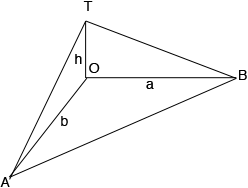SEARCH HOMEMath Central Quandaries & QueriesTwo observers on level ground position themselves 50 meters apart so that an obelisk is due north of position A and due west of position B. The angle of elevation to the top of the obelisk at position A and B is measured to be 29.9 degrees and 37.5 degrees respectively. Use this information to estimate the height of the obelisk.Hi,

In my diagram O is the base of the obelisk and T is the top and the distance from A to B is 50 meters. The distance from O to B is $a$ meters, the distance from O to A is $b$ meters and the height of the obelisk is $h$ meters.Assuming the obelisk is perpendicular to the ground, angles TOB and AOT are right angles. The measure of angle OBT is 37.5 degrees so use a trig function to write $a$ as a function of $h.$ In a similar way write $b$ as a function of $h.$

O is due north of A and O is due west of B and thus BOA is a right triangle. What does Pythagoras theorem tell you?

PennyMath Central is supported by the University of Regina and the Imperial Oil Foundation.